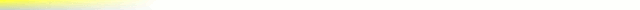# [mkgmap-dev] Is height: filter working as described?

From Carlos Dávila cdavilam at orangecorreo.es on Thu Feb 2 09:45:02 GMT 2017

```According to style manual the rule
natural=peak {name '\${name|def:}\${ele|height:m=>ft|def:}' } [0x6616
resolution 24]
from default style should convert ele values from meters to feet,
assuming ele is in meters, but this is what map shows for the following
ele values:
ele=500, map: ", 500 m"
ele=500m, map: ", 500 m"
ele=500ft, map: ", 152 m"
So it seems to be doing right the opposite, converting from feet to
meters. Also, with m=>ft rule, map should show ft units, not m.
If I invert the rule from m=>ft to ft=>m this is what happens:
ele=500, map: ", 46 m"
ele=500m, map: ", 152 m"
ele=500ft, map: ", 46 m"
Now it seems when values are in feet conversion factor is applied twice.
It seems when height: is used it always does a ft to m conversion first
and then it applies what is specified in the rule.
For the same examples, conv: filter gives the right values.
```